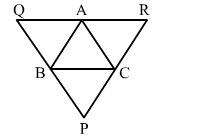# In the adjoining figure, ∆ABC is a triangle and through A, B, C, lines are drawn, parallel respectively to BC,

Question:

In the adjoining figure, ABC is a triangle and through ABC, lines are drawn, parallel respectively to BCCA and AB, intersecting at PQ and R. Prove that the perimeter of ∆PQR is double the perimeter of ∆ABC.Solution:

Perimeter of ∆​ABC = AB + BC + CA                         ...(i)
Perimeter of ∆PQR  =​ PQ + QR + PR                   ...(ii)

BC || QA and CA || QB
i.e., BCQA is a parallelogram.
∴ BC = QA                                  ...(iii)
Similarly, BC || AR and AB || CR
i.e., BCRA is a parallelogram.
∴ BC = AR                                    ...(iv)
But, QR = QA + AR
From (iii) and (iv), we get:
⇒ QR = BC + BC
⇒ QR = 2BC

$\therefore B C=\frac{1}{2} Q R$

Similarly, $C A=\frac{1}{2} P Q$ and $A B=\frac{1}{2} P R$

From (i) and (ii), we have:

Perimeter of $\triangle A B C=\frac{1}{2} Q R+\frac{1}{2} P Q+\frac{1}{2} P R$

$=\frac{1}{2}(P R+Q R+P Q)$

i.e., Perimeter of $\triangle A B C=\frac{1}{2}$ (Perimeter of $\triangle P Q R$ )

$\therefore$ Perimeter of $\triangle P Q R=2 \times$ Perimeter of $\triangle A B C$